Smartick is a fun way to learn math!# I want to learn about: Mathematics

Learn mathematics with Smartick. Exercises, tutorials, problems and teaching resources for children learning elementary mathematics. Here you will find posts about mathematics to make learning easier and more fun.

Apr14

## Sudokus: What are they, and how are they solved?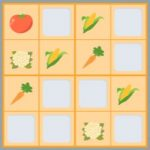Have you ever tried to solve a Sudoku? Sudokus are one of the most popular pastimes in the world. These puzzles require logic and problem-solving skills, so they can help you develop your maths skills. Let’s learn how to play Sudoku and some tips on how to solve them! What are sudokus? Traditionally, sudokus are […]

Mar24

## Algebra Problems: 3 Types of Activities in Smartick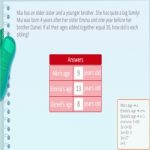Algebra is a branch of mathematics that focuses on the study of mathematical structures and the operations that can be performed with them. In particular, algebra focuses on the use of symbols and letters to represent numbers and mathematical expressions, making it possible to work with them in a general and abstract way. In algebra, […]

Mar03

## How to Calculate the Area?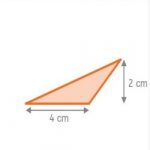Geometry is seen in school as a collection of formulas that answer the question, how to calculate the area? Geometry is much more than that: it comprises the whole part of mathematics that solves problems of space, answers questions about the shape of plane and three-dimensional figures and, it goes much further, trying to answer […]

Feb16

## 5 Math Activities for Preschoolers with ExamplesIn this post we will see some examples of the math activities for preschoolers that we have in Smartick. Students of this age will be exposed to a fully customized sequence of activities that will allow them to develop different math skills. Introduction: math activities for preschoolers Smartick is an online program that offers personalized […]

Feb09

## Geometric Shapes with ExamplesToday you will learn about geometric shapes. We are going to teach you about the characteristics of the main geometric shapes, and to help you understand better, we will explain with examples. What Are Geometric Shapes? The ancient Greeks defined straight and curved lines by looking at their environment. Do you think they stopped there? […]

Dec31

## Addition With and Without Carrying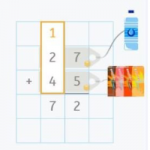In today’s post, we are going to learn how to solve addition problems with and without carrying. We will also see some examples of exercises that children complete in Smartick. But first, let’s review what addition is! What is Addition? Addition is the act of adding, joining, or putting elements together. When we perform this […]

Aug31

## Direct and Inverse Proportionality ProblemsIn today’s post, we are going to work on proportionality by looking at some examples of proportionality problems. Before we begin, you can take a look at other exercises with proportional numbers where we review the concepts of reason and proportionality constant. Direct Proportionality and Inverse Proportionality Proportional magnitudes can be directly proportional or inversely […]

Jul23

## Mental Math Strategies for Children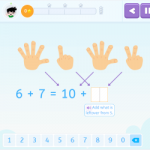Scientific evidence tells us that children with learning difficulties who do not use mental math strategies have poor results in this area, similar to those of children with learning difficulties specific to math. That is why at Smartick we wanted to make sure that all children develop mental math strategies. In this post, we are […]

May05

## Half, Third, Fourth, Fifth in Math: Definition & CalculationIn this post, we are going to learn how to calculate a half, third, fourth, and fifth. These expressions are not just used for math problems, but also in daily life! Do you know what they are? Do you know how to calculate them? You are going to see how easy it is to calculate […]

Apr21

## Subtraction With and Without CarryingToday we are going to learn how to solve subtraction problems both with and without carrying. We will begin with a review of what subtraction is and its elements, and then we will see an example of each type of subtraction with a video. What is Subtraction? Subtraction is a mathematical operation that consists of […]

Apr13

## What is Multiplication: Steps to Learn and Understand Multiplication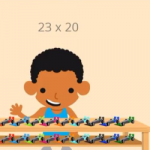What is multiplication? We will explain it in this post! Then we will show some examples using the steps that you should follow to complete multiplication problems, along with a video. What is Multiplication? Multiplication is a mathematical operation that is performed to calculate the result by adding the number as many times as indicated […]